Courses

# Environmental Engineering - 2

## 10 Questions MCQ Test GATE Civil Engineering (CE) 2022 Mock Test Series | Environmental Engineering - 2

Description
This mock test of Environmental Engineering - 2 for Civil Engineering (CE) helps you for every Civil Engineering (CE) entrance exam. This contains 10 Multiple Choice Questions for Civil Engineering (CE) Environmental Engineering - 2 (mcq) to study with solutions a complete question bank. The solved questions answers in this Environmental Engineering - 2 quiz give you a good mix of easy questions and tough questions. Civil Engineering (CE) students definitely take this Environmental Engineering - 2 exercise for a better result in the exam. You can find other Environmental Engineering - 2 extra questions, long questions & short questions for Civil Engineering (CE) on EduRev as well by searching above.
QUESTION: 1

### The effective size of the particle (D10) of filter [slow sand filter] is

Solution:

As per Government of India’s Effective size (D10) of filter for slow sand filter is in the range of (0.2 to 0.3) mm and Coefficient of uniformity for this filter media is in the range of (3 – 5)

QUESTION: 2

### Zero hardness of water is achieved by

Solution:

Water of zero hardness can be obtained from ion exchange method only. It is also called zeolite process or base exchange treatment.

QUESTION: 3

### The cleaning of slow sand filter is done by –

Solution:

The cleaning of slow sand filter is done by scrapping off the top sand layer and cleaning of rapid sand filter and pressure filter is done by passing air and by back - washing.

QUESTION: 4

The efficiency of sediment removal in a continuous flow sedimentation tank does not depend upon-

Solution:

For particle removal, setting velocity > Surface loading rate.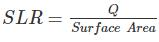Settling velocity is depended upon the particle size & SLR is on the surface area which depends on length and width.

So efficiency of particle removal does not depend upon the depth of the tank.

QUESTION: 5

The design parameter for flocculation is given by a dimensionless number Gt, where G is the velocity gradient and t is the detention time. Values of Gt ranging from 104 to 105 are commonly used, with t ranging from 10 to 30 min. the most preferred combination of G and t to produce smaller and denser flocs is

Solution:

Large G values with short times tend to produce small, dense flocs, while low G values and long times produce large, lighter flocs. Since large, dense flocs are more easily removed in the settling basin, it may be advantageous to vary the G values over the length of the flocculation basin. The small dense flocs produced at high G values subsequently combine into larger flocs at the lower G values. Reduction in G values by a factor of 2 from the influent end to the effluent end of the flocculator has been shown to be effective.

QUESTION: 6

A circular primary clarifier processes an average flow of 5005 m3/d of municipal wastewater. The overflow rate is 35 m3/m2/d. the diameter of clarifier shall be

Solution:

Area of clarifier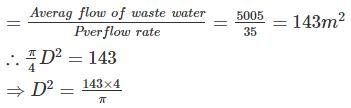⇒ D = 13.5 m

QUESTION: 7

Match List – I (Type of water impurity) with List – II (Method of treatment) and select the correct answer using the codes given below the lists: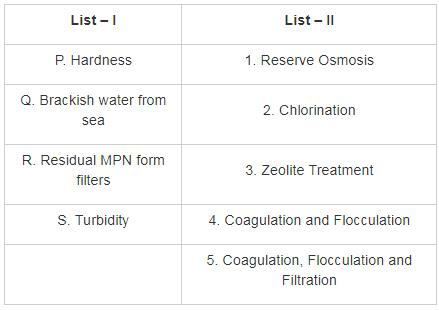Solution: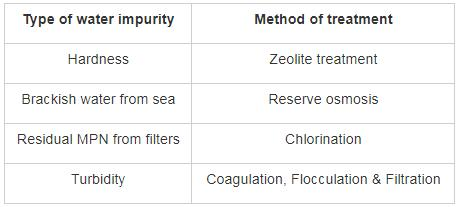QUESTION: 8

For water, the dosage at a breakpoint is 1.5 mg/L and residual chlorine at that time is found to be 0.3 mg/L. If cumulative chlorine added is 2 mg/L, the total residual chlorine will be –

Solution:

Break point chlorination = Chlorine demand = 1.5 mg/L

Combined Cl2 residual at that time = 0.3 mg/L

Chlorine dosage = 2 mg/L

∴ Free chlorine residual = Dose – demand

= 2 – 1.5

= 0.5 mg/L

∴ Total chlorine residual = Free chlorine residual + combined chlorine residual

= 0.5 + 0.3

= 0.8 mg/L

*Answer can only contain numeric values
QUESTION: 9

A water treatment plant is required to process 50,000 m3 water per day. The rapid mixing tank will blend 50 mg/L of alum with the flow and have a detention time of 2 min. The power input (in kilowatt) necessary for a G value of 750 S-1 at water temperature 22°C is _____.

(use kinematic viscosity at 22°C as 1 × 10-6 m2/s)

Solution:

Power input (P) = μVG2

Where, μ = dynamic viscosity of water

V = volume of the tank

G = velocity gradient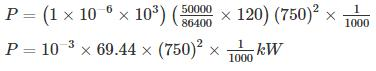P = 39.0625 kW

QUESTION: 10

A channel-type grit chamber has a flow-through velocity of 0.15 m/s, a depth of 0.8 m and a length of 10 m. For inorganic particles with the specific gravity of 2.7, and considering kinematic viscosity of 8×10-7 m2/s the largest diameter that can be removed with 95% efficiency is

Solution:

The settling velocity can be determined through the equation,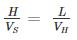where H = depth of the tank, L = length of the tank, Vs  = Settling velocity, and VH = Flow through velocity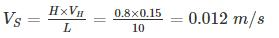Now, using Stokes law,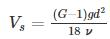where G = specific gravity of the particle, ν =kinematic viscosity of the particle, g = acceleration due to gravity, d = diameter of the particle

For 95% removal efficiency,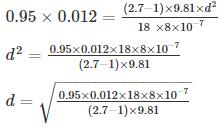d = 9.92 × 10−5m
d = 0.0992.mm